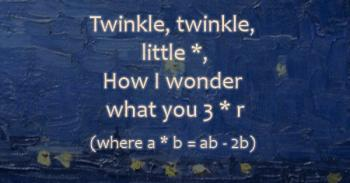Games
Problems
Go Pro!

Vera from Michigan asks, "What is a star operation?"First, let's talk for a second about operations in general. Operations are things that we can do to one or more expressions in order to create a new value from them. I say "one or more," because it's possible to have an operation that works on a single expression; we call that a unary operator. Most operations that you're familiar with take two expressions and combine them to make a new expression. We call these binary operators. In this blog post we'll talk about binary operators, and maybe someday I'll come back and do a blog post about unary operators.

What are some examples of binary operators? Multiplication is a good example:

3 x 5 = 15

In this example, we took two expressions (3 and 5) and we used the multiplication operator (x, or •) to combine them to create a new value:15. Now, you know that 3 x 5 = 15 because you memorized it at some point, but it wasn't just arbitrary memorization that got our result; the operation has a rule that gives you the result. The rule can be expressed in a few ways - here are just a couple:

• count to 3, five times. How many numbers did you count?
• create a rectangle that's 3 units wide and 5 units long. What is the area of the box in square units?

Every operation has a rule, or pattern, that we use to combine the two expressions into a single expression.

What are some other operators you're familiar with? Addition (+), subtraction (-), division (/).

But what is a star operation? Well, math teachers get bored, sometimes, with so few operations to confuse their students with, so they feel like inventing their own operations, complete with their own rules and their own symbols. Since the asterisk isn't used as an operation symbol (except in computer programming), we often use the asterisk as our operation symbol. Since the asterisk looks like a star, we dubbed it a "star operation." Let's look at an example:

Example One
We'll define our rule as follows a * b = a2 + b2.

This means that if we see something like 3 * 5, we'll plug the 3 in place of the a, and 5 in place of the b:

3 * 5 = 32 + 52 = 9 + 25 = 34.

Example Two
Rule: a * b = a(b - 1)

Find the value of 4 * 7.

In this case, we'll plug the 4 into our rule in place of a, and the 7 in place of the b:

4 * 7 = 4(7 - 1) = 4(6) =24.

By the way, it's interesting to note that our first example is commutative, which means that a * b = b * a, but the second rule is not. If we calculate 7 * 4 instead of 4 * 7, we don't get the same result:

7 * 4 = 7(4 - 1) = 7(3) = 21.

We don't have to use the asterisk symbol for our operation, by the way. We can pick any other unused symbol we want. It's not unusual to see a delta symbol (the Greek letter delta is a triangle with one vertex at the top) or an inverted delta symbol (pointing down instead of up), or a square, or a diamond, etc. We still often refer to them as star operations, even if they don't use the asterisk symbol.

Example Three
Rule: a ♦ b = (a + b)/2

Find the value of (2 ♦ 4) ♦ 6.

Notice that in this problem, we have parentheses, and our standard order of operations applies here - we have to evaluate the part in parentheses first:

2 ♦ 4 = (2 + 4)/2 = 6/2 = 3.

Now we evaluate 3 ♦ 6:

3 ♦ 6 = (3 + 6)/2 = 9/2 = 4.5.

As a side note, we observe that this operation is not associative; if we shift the parentheses, we get a different result: 2 ♦ (4 ♦ 6) = 3.5.

In closing, it can be fun to try to guess the rule for a star operation, given a table of values. Take a look at this example:

5 ¤ 3 = 16
7 ¤ 4 = 33
8 ¤ 6 = 28
8 ¤ 2 = 60

What do you think the rule could be? There could be more than one possible answer, and I'll leave it to you to figure it out. I'll give you one more equation to test your rule against:

1 ¤ 7 = -48

# Blogs on This SiteReviews and book lists - books we love!The site administrator fields questions from visitors.Like us on Facebook to get updates about new resources